Next: The likelihood term Up: Evaluating the cost function Previous: Evaluating the cost function   Contents

### The terms originating from the parameters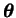Assuming the parameters are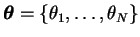and the approximation is of the form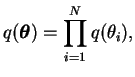(6.2)

the terms of Equation (6.1) originating from the parameterscan be written as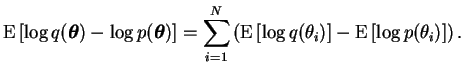(6.3)

In the case of Dirichlet distributions one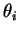in the previous equation must of course consist of a vector of parameters for the single distribution.

There are two different kinds of parameters in, those with Gaussian distribution and those with a Dirichlet distribution. In the Gaussian case the expectation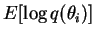over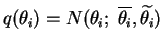gives the negative entropy of a Gaussian,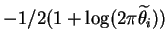, as derived in Equation (A.5) of Appendix A.

The expectation of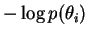can also be evaluated using the formulas of Appendix A. Assuming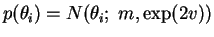(6.4)

where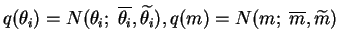and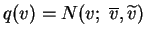, the expectation becomes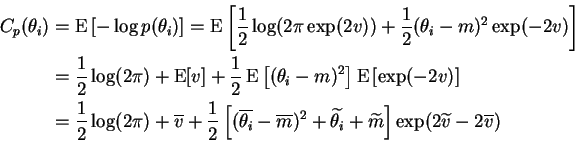(6.5)

where we have used the results of Equations (A.4) and (A.6).

For Dirichlet distributed parameters, the procedure is similar. Let us assume that the parameter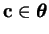,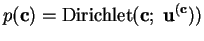and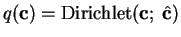. Using the notation of Appendix A, the negative entropy of the Dirichlet distribution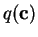,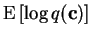, can be evaluated as in Equation (A.14) to yield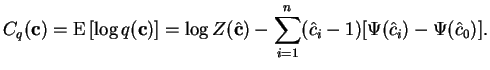(6.6)

The special function required in these terms is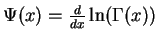, where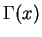is the gamma function. The psi function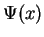is also known as the digamma function and it can be efficiently evaluated numerically for example using techniques described in . The term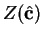is a normalising constant of the Dirichlet distribution as defined in Appendix A.

The expectation of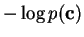can be evaluated similarly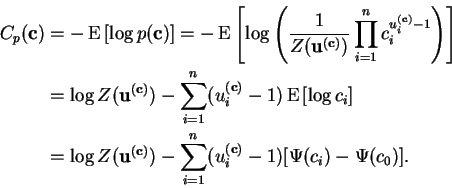(6.7)Next: The likelihood term Up: Evaluating the cost function Previous: Evaluating the cost function   Contents
Antti Honkela 2001-05-30